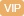| APP | 校园号 | 客服 客服热线：400-863-9889

400-863-98896
6

31×5=155
31×5=155
【考点】最大与最小【专题】运算顺序及法则．

【分析】把64化成8，再用乘法的交换律、结合进行算；

【解答】解：64×1255
=10×101
=720；
=（8×12）（×8）
4002000
=400；
=1101+8118+18
30+72+98）+1271
=54×101
=30+98）+271+729）
=0000-880
2×404
=15×80-3×80
118+81×1+18
=8×155×8
=8（10118+1）
8×8×1255
=210．
【点评】此题查目的是掌握整数算定律，并且能够灵活运用这些定律运算性进简便计算．

0/0